Learning Library

Dizzy Over Decimals!

Decimals can be a drag when you're just starting out with them. Introduce your 4th grader to these decimal worksheets, and pretty soon she'll have no trouble at all dividing and conquering decimals.

Click on a worksheet in the set below to see more info or download the PDF.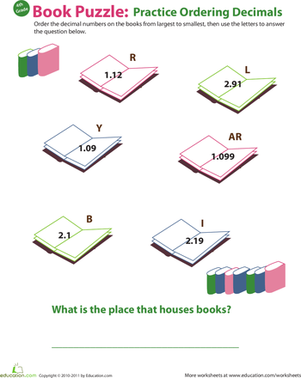Decimal Dash: Practice Ordering Decimals #3

Encourage your fourth grader to dive into decimals with this bookish math worksheet. In this worksheet he'll order decimals from greatest to least.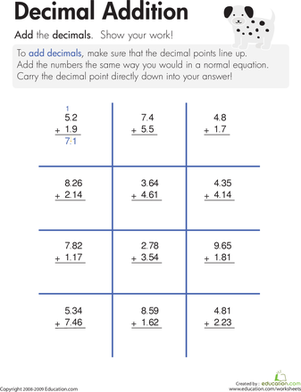Let's get to the point: the decimal point, that is! In this worksheet, children practice adding decimals.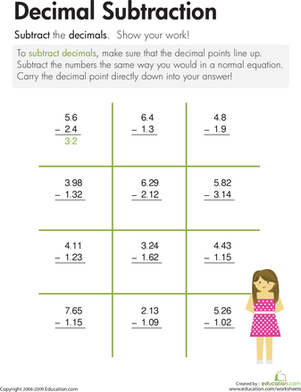Decimal Subtraction

Let's get to the point: the decimal point, that is! On this third grade math worksheet, kids learn how to subtract decimals.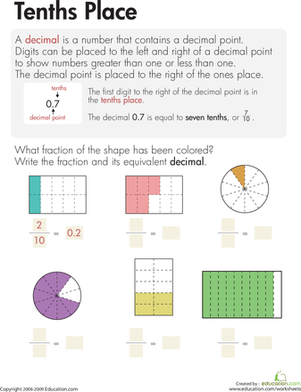Decimals: Tenths Place

This third grade math worksheet helps kids understand tenths and that fractions and decimals are two different ways to show the same concept.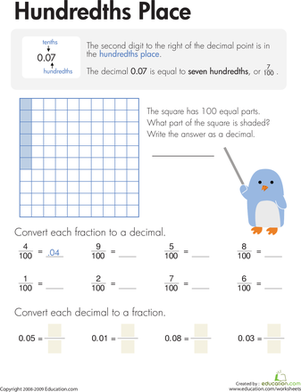Decimals: Hundredths Place

This third grade math worksheet helps kids understand hundredths and that fractions and decimals are two different ways to show the same concept.Fractions & Decimals: Lock & Key

Practice is one of the keys to mastering concepts, and this third grade math worksheet offers great practice with equivalent fractions and decimals.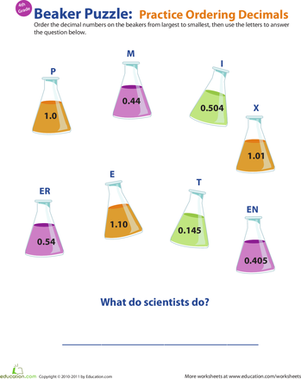Decimal Dash: Practice Ordering Decimals #1

Does your fourth grader need help with ordering decimals? Bolster his decimal understanding with this beaker-booked worksheet.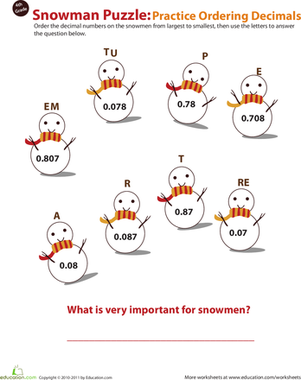Decimal Dash: Practice Ordering Decimals #2

Give your fourth grader's math skills a hand with our decimal worksheet. Snowmen keep him company as he practices ordering decimal numbers from big to small.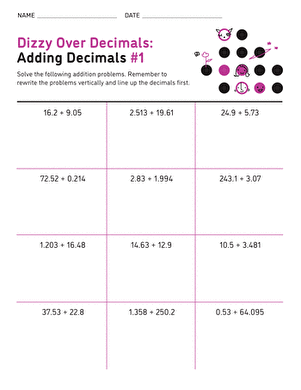Dizzy Over Decimals: Addition #1

Adding up decimals is as simple as 1, 2, 3. Your kid will get comfortable adding up decimals in no time!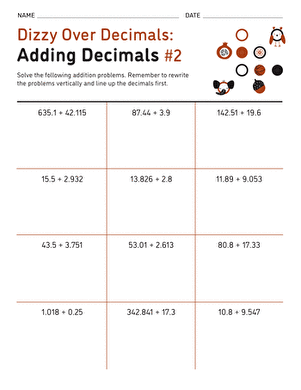Dizzy Over Decimals: Addition #2

Once your kid has gotten comfortable adding up multi-digit numbers, it's time to dive into decimals!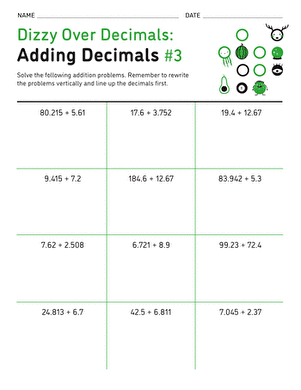Dizzy Over Decimals: Addition #3

If adding up decimals is getting your child down, then we've got the worksheet for her!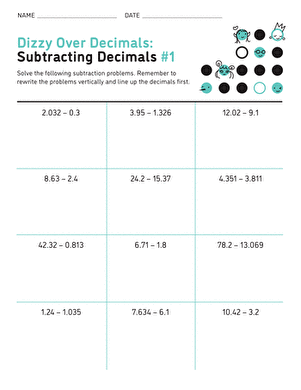Dizzy Over Decimals: Subtraction #1

This math practice sheet gives your kid the help he needs to be cool, calm, and collected when it comes to subtracting decimals.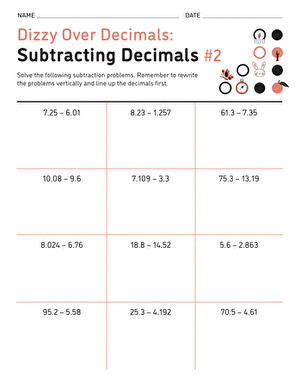Dizzy Over Decimals: Subtraction #2

Teach your child how to subtract decimals; it's easy easy as 1, 2, 3!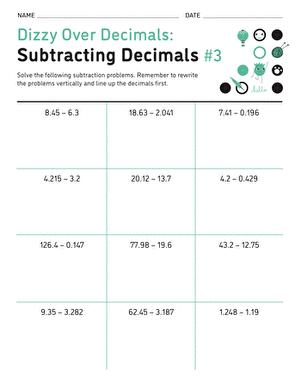Dizzy Over Decimals: Subtraction #3

Here's an easy way to ease your kid's concerns about subtracting decimals: practice, practice, and more practice!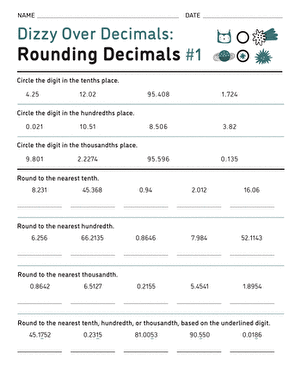Dizzy Over Decimals: Rounding #1

Does your child need a little extra practice with decimal place values? This worksheet is the way to go.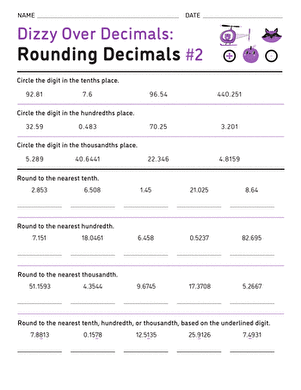Dizzy Over Decimals: Rounding #2

Is your child getting dizzy over decimals? This worksheet is sure to give her all the practice she needs to get comfortable with decimal place values.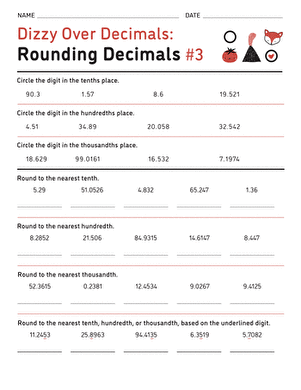Dizzy Over Decimals: Rounding #3

This worksheet is a great way to ensure your child understands decimal place value before introducing him to more complicated problems.

Want to download this whole set as a single PDF?

Create new collection

0

New Collection>

0 items

What could we do to improve Education.com?

Please note: Use the Contact Us link at the bottom of our website for account-specific questions or issues.

What would make you love Education.com?